## Table of ContentOpen Access

ARTICLE

# Design of a Computational Heuristic to Solve the Nonlinear Liénard Differential Model

Li Yan1, Zulqurnain Sabir2, Esin Ilhan3, Muhammad Asif Zahoor Raja4, Wei Gao5, Haci Mehmet Baskonus6,*

1 School of Engineering, Honghe University, Mengzi, 661199, China
2 Department of Mathematics and Statistics, Hazara University, Mansehra, 21300, Pakistan
3 Faculty of Engineering and Architecture, Kirsehir Ahi Evran University, Kirsehir, 40500, Turkey
4 Future Technology Research Center, National Yunlin University of Science and Technology, Yunlin, 64002, Taiwan
5 School of Information Science and Technology, Yunnan Normal University, Kunming, 650500, China
6 Department of Mathematics and Science Education, Faculty of Education, Harran University, Sanliurfa, 63500, Turkey

* Corresponding Author: Haci Mehmet Baskonus. Email:Computer Modeling in Engineering & Sciences 2023, 136(1), 201-221. https://doi.org/10.32604/cmes.2023.025094

## Abstract

In this study, the design of a computational heuristic based on the nonlinear Liénard model is presented using the efficiency of artificial neural networks (ANNs) along with the hybridization procedures of global and local search approaches. The global search genetic algorithm (GA) and local search sequential quadratic programming scheme (SQPS) are implemented to solve the nonlinear Liénard model. An objective function using the differential model and boundary conditions is designed and optimized by the hybrid computing strength of the GA-SQPS. The motivation of the ANN procedures along with GA-SQPS comes to present reliable, feasible and precise frameworks to tackle stiff and highly nonlinear differential models. The designed procedures of ANNs along with GA-SQPS are applied for three highly nonlinear differential models. The achieved numerical outcomes on multiple trials using the designed procedures are compared to authenticate the correctness, viability and efficacy. Moreover, statistical performances based on different measures are also provided to check the reliability of the ANN along with GA-SQPS.

## Keywords

1  Introduction

In scientific fields, most of the real-world problems are observed in plasma physics, solid state physics, mathematical biology, fluid dynamics, and chemical kinetics, which have been stated in the form of differential systems . Various approaches have been exploited to solve these types of models, like Chebyshev polynomial based approximation scheme, closed-form scheme, spectral collocation method, subdomain finite element scheme, Bernoulli collocation method, predictor–corrector scheme, variational iteration scheme, homotopy perturbation scheme, differential quadrature scheme, quantic B-spline differential scheme, power series scheme, differential transformation scheme, Pade series scheme and Legendre polynomial function approximation and so on .

The present study is related to solving a nonlinear Liénard model (NLM) using the efficiency of artificial neural networks (ANNs) along with hybridization procedures of global search genetic algorithm (GA) and local search sequential quadratic programming scheme (SQPS). All the above-cited schemes have their own benefits and drawbacks, however, the stochastic computing efficiency based on ANN along with GA-SQPS is neither been tested nor implemented to solve the stiff-natured NLM. The NLM is mathematically given as :

{f(Ω)+q(f)f(Ω)+u(f)=g(Ω),f(Ω0)=A0,f(Ω0)=A1.(1)

The above form of the NLM is used to generalize the damped springmass system as well as these models have been applied in the physically substantial areas by taking different values of the q(f), u(f) and g(Ω). In the above NLM, u(f) is the restoring force, g(Ω) is the external force and q(f)f(Ω) is the damping force. By taking q(f)=ε(f21), u(f)=f and g(Ω)=0, the model form becomes the nonlinear Van der Pol model based on the electronic vacillation . The NLM is implemented in the modeling of fluid mechanics . Furthermore, the vacuum tube technology progressed by using oscillating circuits based on the NLM .

Due to the huge importance of the NLM, the focus of the research community was to present the significant applications of this model . The computing stochastic solvers are used to present the solutions of various singular models, functional differential systems, biological systems, prediction models and fractional applications . However, the stochastic computing efficiency based on the strength of ANN along with GA-SQPS has not been implemented to present the numerical performances based on the NLM. These stochastic contributions proved the worth to observe the robustness and accuracy-based ANN along with GA-SQPS for solving the NDM.

Few novel influences based on the stochastic scheme are shortened as:

•   Design of ANN along with GA-SQPS is presented successfully for solving the NLM.

•   The matching of achieved and exact results for three different problems based on the NLM establish convergence, reliability and precision.

•   The values of the absolute error (AE) are obtained in good measures for solving each example based on the NLM.

•   The consistent, accurate performance is observed through statistical observations on multiple trials of ANN along with GA-SQPS in terms of semi-interquartile range (S.I.R), mean absolute deviation (MAD), Nash Sutcliffe Efficiency (NSE) and Theil’s Inequality Coefficient (TIC) metrics.

•   Beside sensibly precise continuous input training interval, ease in the concept, robustness, smooth implementable training, extendibility and stability are other commendable proclamations for the designed ANN along with GA-SQPS.

The rest of the paper is organized as; Section 2 presents the designed procedure, optimization process and statistical performances. Section 3 describes the discussions of the results. Section 4 shows the conclusions and upcoming research directions.

2  Methodology

This section presents the design of the ANN along with GA-SQPS measures to solve the nonlinear Liénard differential model. The detail of fitness function is accessible using the mean square error along with the learning practice of the hybrid computing GA-SQPS.

2.1 Mathematical ANN Modeling

The ANNs have been exploited to solve various applications arising in numerous fields . The proposed results are signified as f^(Ω), while f^(n)(Ω) is the nthderivative. The mathematical terminologies are given as:

f^(Ω)=P=1kaPL(wPΩ+bP),(2)

f^(n)(Ω)=P=1kaPL(n)(wPΩ+bP),

where k signifies the neurons, L is the activation function and [aP,wP,bP] denote the pth component form of [a,w,b]. The log-sigmoid L(Ω)=1/(1+eΩ) activation function in the networks is written as:

f^(Ω)=P=1kaP(11+e(wPΩ+bP)),(3)

f^(Ω)=P=1kaPwP(e(wPΩ+bP)(1+e(wPΩ+bP))2),

f^(n)(Ω)=P=1kaPwP(e(wPΩ+bP)(1+e(wPΩ+bP))n+1e(n+1)(wPΩ+bP)(1+e(wPΩ+bP))n).

An error based fitness function (EFit) is given as:

EFit=EFiti+EFitii.(4)

Here EFiti and EFitii are the fitness functions associated to NLM and its boundary conditions, given as:

EFit=1NP=1N(f+q(fP)fP+u(fP)gP)2+12((f^0A0)2+(f^0A1)2),(5)

where Nh=1,fP=f(Ω),q(f)=q(fP),U(f)=U(fP) and gP=gh. One may describe the Eq. (1) with the availability of suitable weights W, i.e., EFit0.

2.2 Optimization Procedure: GA-SQPS

The optimization processes to solve the nonlinear Liénard differential model using the designed ANN along with GA-SQPS measures are provided in this section.

GA is an optimization based global search technique work to present the solutions of the linear and nonlinear networks. It is normally implemented to regulate the precise population results for numerous steep/complex systems based on the optimal training performance. GA is applied recently as automatic clustering , molecular sequence alignment, bioinformatics , energy efficiency and thermal comfort in building design , structural optimization , weight setting problem in OSPF/IS-IS routing , assembly sequences , image annotation , CNN template learning  and risk-based partner selection problem .

The performance based on the GA optimization converges rapidly by the hybridization with the local search scheme by taking its best values as initial inputs. Therefore, an efficient SQPS, i.e., a local search technique is implemented in the process of hybridization. SQPS is used recently in unified full-chip CMP model aware dummy fill insertion framework , quasilinear parabolic optimal control problems , convex/nonconvex economic dispatch models , fractional order control design  and economic dispatch of micro-grid  and some related other applications . The designed structure using the ANN procedures along with GA-SQPS for the NLM is presented in Fig. 1.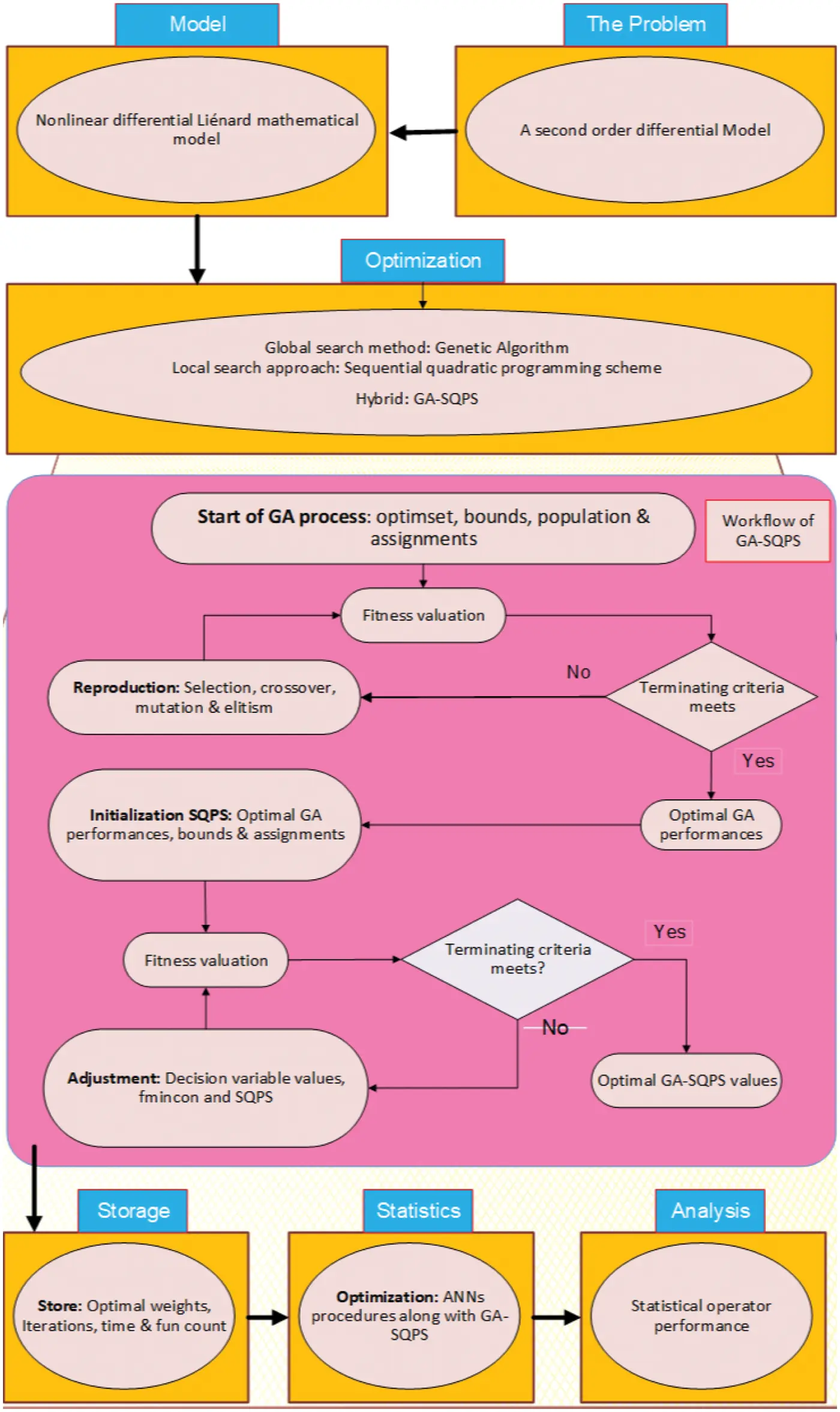Figure 1: Designed structure using the ANN procedures along with GA-SQPS for the NLM

2.3 Performance Measures

The statistical operators performances of MAD, TIC, S.I.R and ENSE are provided together with the global representations to check the reliability of the designed ANN along with GA-SQPS. The detailed pseudocode based structure of the designed ANN along with GA-SQPS for solving the NLM is presented in Table 1. The mathematical notations of the statistical operators are written as:

TIC=1nr=1n(frf^r)2(1nr=1nfr2+1nr=1nf^r2),(7)

{S.I.R=12×(q1q3),q1=1stquartile&q3=3rd quartile,(8)

NSE={1r=1n(frf^r)2(frf¯r)2,f¯r=1nr=1nfr,(9)

ENSE =1NSE.(10)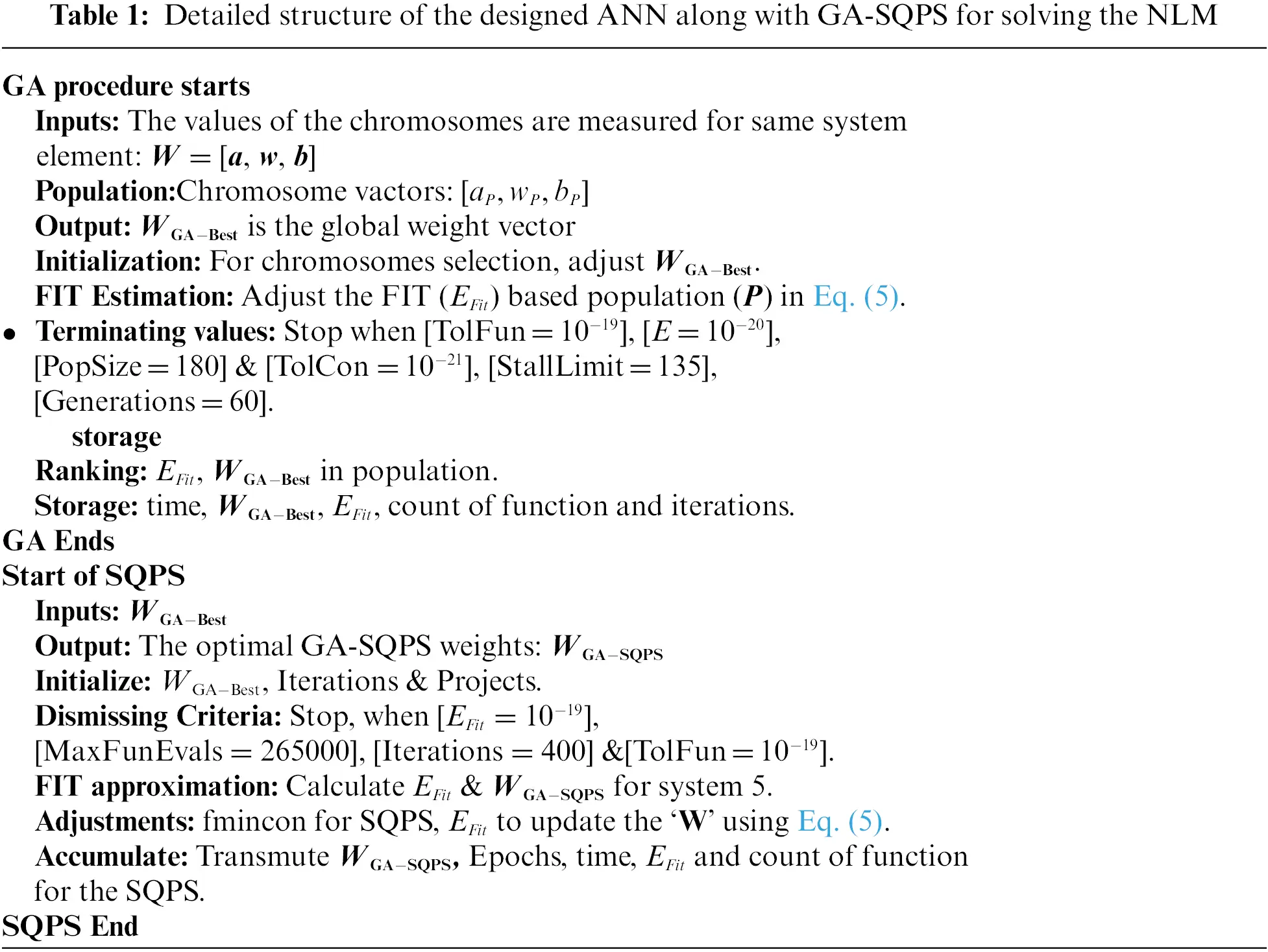3  Simulations and Results Performance

The current section present the result discussions for three different problems based NLM by applying the ANN along with GA-SQPS. The graphical and numerical measures have been provided to assess the convergence and accuracy.

Problem 1: Consider the NLM involving trigonometric ratios is given as :

{f(Ω)+f(Ω)f(Ω)+f(Ω)+f2(Ω)=cos2(Ω)cos(Ω)sin(Ω),f(0)=1,f(0)=0.(11)

The exact form of the solution is cos(Ω), the error function is given as:

EFit=1Nm=1N(f^p+f^pf^p+f^p+f^p2cos2(Ωp)+sin(Ωp)cos(Ωp))2+12((f^p1)2+(f^p)2).(12)

Problem 2: Suppose the higly NLM is written as :

{f(Ω)f(Ω)+4f2(Ω)3f5(Ω)=0,f(0)=(2)1,f(0)=24.(13)

The exact solution of Eq. (13) is 1+tanh(Ω)2 and the error function is given as:

EFit=1Nm=1N(f^pf^p+4f^p23f^p5)2+12((f^0(2)1)2+(f^024)2).(14)

Problem 3: Suppose the higly NLM is shown as :

{f(Ω)f(Ω)+4f2(Ω)+3f5(Ω)=0,f(0)=(1+2)1,f(0)=0.(15)

The exact solution of Eq. (13) is sech2(Ω)/((12)sech2(Ω)+22) and the error function is given as:

EFit=1Nm=1N(f^pf^p+4f^p2+3f^p5)2+12((f^0(1+2)1)2+(f^0)2).(16)

The results based on NLM for problems 1 to 3 have been performed through the numerical performance of global and local search GA-SQPS. These optimization procedures of GA-SQPS is provided in Table 1 and the whole practice is repeated for fifty multiple runs to produce a larger data of parameters. The proposed results through the designed ANN along with GA-SQPS are provided using the trained weights and mathematically given as:

f^P1(Ω)=1.62601+e(1.3507Ω0.980)1.89111+e(1.4324Ω+1.803)0.02661+e(0.1345Ω0.554)0.47611+e(0.880Ω0.7503)2.49291+e(1.3578Ω2.348)0.86641+e(0.4878Ω1.674)+0.63851+e(1.0891Ω0.0251)0.02471+e(1.138Ω+0.8001)0.40661+e(1.049Ω2.011)0.92071+e(1.0495Ω2.011),(17)

f^P2(Ω)=0.99061+e(0.305Ω+2.876)1.21101+e(0.981Ω0.291)+1.00461+e(0.798Ω6.839)0.05691+e(7.448Ω+11.626)0.60151+e(14.55Ω+10.770)15.27971+e(0.210Ω11.497)+1.99421+e(0.820Ω+1.210)0.50411+e(2.929Ω3.345)0.23041+e(8.494Ω+8.144)+0.79761+e(1.361Ω+1.195),(18)

f^P3(Ω)=1.82761+e(0.135Ω1.29624)0.28991+e(0.263Ω1.609)2.59101+e(1.609Ω3.645)0.06041+e(1.162Ω3.645)1.58691+e(0.031Ω+0.5801)1.72961+e(2.14Ω+0.087)+0.11341+e(0.099Ω+0.378)0.93851+e(1.210Ω0.7525)+0.49301+e(0.085Ω1.0692)1.91951+e(2.25Ω0.339).(19)

The graphical measures are provided in Figs. 2 to 6 for each problem based on a nonlinear Liénard differential model using 30 numbers of variable, 10 neurons with [0,1] input and 0.05 step size. The obtained results based on best weights are given in Figs. 2a2c using the above Eqs. (17)(19). The comparison plots based on the exact, best and mean solutions are accessible in Figs. 2d2f. The mean and best results are plotted using the proposed ANN along with GA-SQPS and presented with the true results. The matching of the proposed and true outcomes validates the correctness of the proposed ANN along with GA-SQPS. The AE plots based on the best and mean solutions are drawn in Subfigures 2g and 2h. It is observed that the AE best values for problems 1–3 lie 10−07 to 10−10, 10−05 to 10−07 and 10−06 to 10−08, respectively. The mean AE values were found around 10−02–10−04, 10−03–10−04 and 10−02–10−03 for problems 1, 2 and 3. The performance procedures for the best, mean and worst results for the NLM is provided in Figs. 2j2l. For problem 1, the best MAD, TIC and ENSE operator values lie around 10−07 to 10−10, 10−10 to 10−11 and 10−13–10−15, the values based on the mean operators of MAD, TIC and ENSE lie as 10−03–10−05, 10−06–10−08 and 10−03–10−04, while the worst MAD, TIC and ENSE operators found as 10−01–10−02, 10−04–10−05 and 10−02–10−03. For 2nd problem, the best MAD, TIC and ENSE operators are calculated as 10−05–10−06, 10−08–10−09 and 10−04–10−05, the MAD, TIC and ENSE operator mean values are 10−03–10−04, 10−07–10−08 and 10−02–10−04, while the MAD, TIC and ENSE worst operator measures found as 10−02–10−03, 10−05–10−06 and 10−03–10−04. For 3rd problem, the best MAD, TIC and ENSE operators found as 10−05–10−06, 10−09–10−10 and 10−10–10−11, the MAD, TIC and ENSE mean operators are 10−03–10−04, 10−06–10−08 and 10−02–10−04, while the MAD, TIC and ENSE operator worst performances found as 10−01–10−02, 10−03–10−05 and 10−02–10−03. These best presentations through the comparison of the results in the form of AE along with the statistical operators indicate the correctness of the proposed scheme for the NLM.Figure 2: Best weights, solution plots, AE and performance indices for each problem of NLM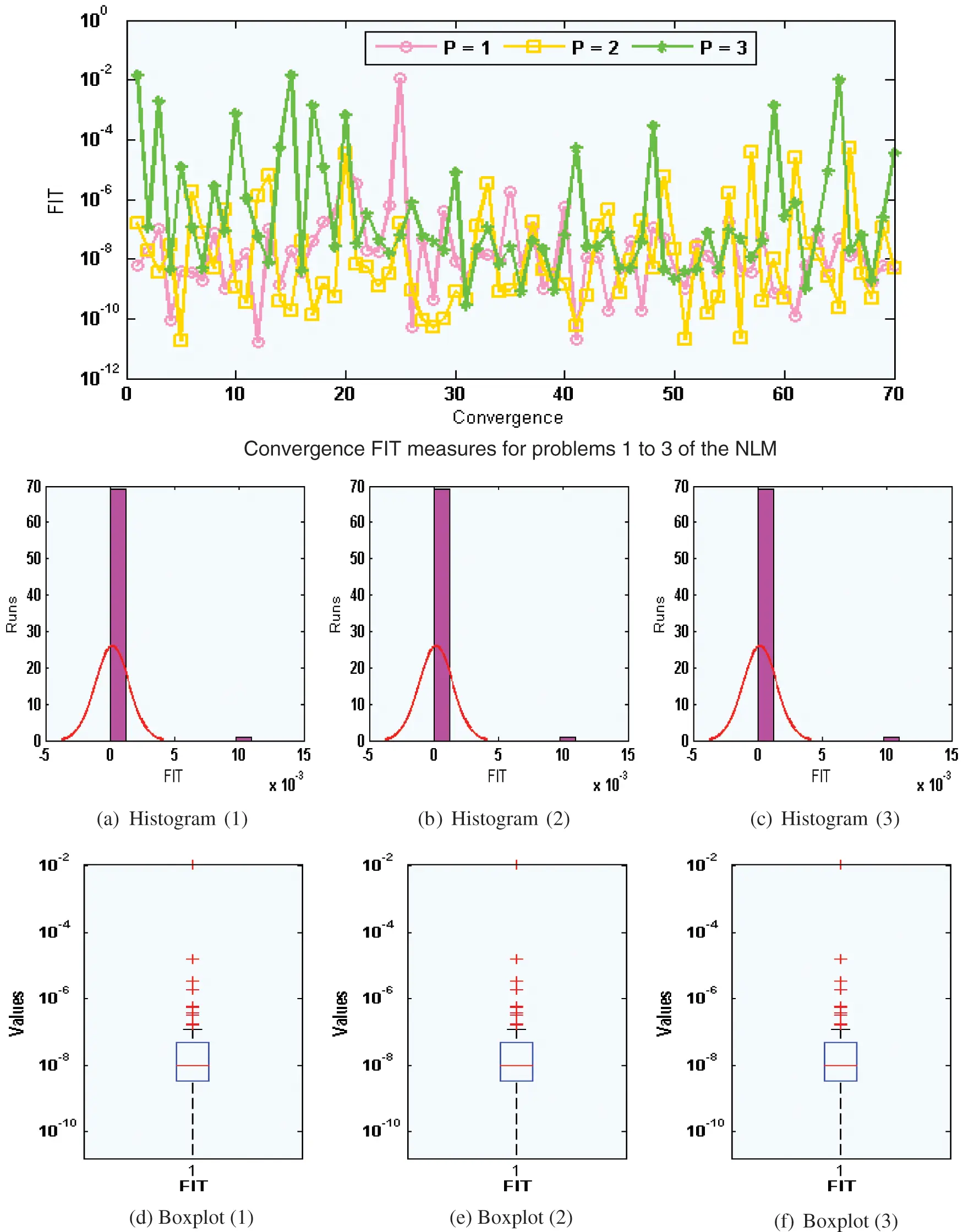Figure 3: Performances of the FIT convergence for problems 1 to 3 based NLM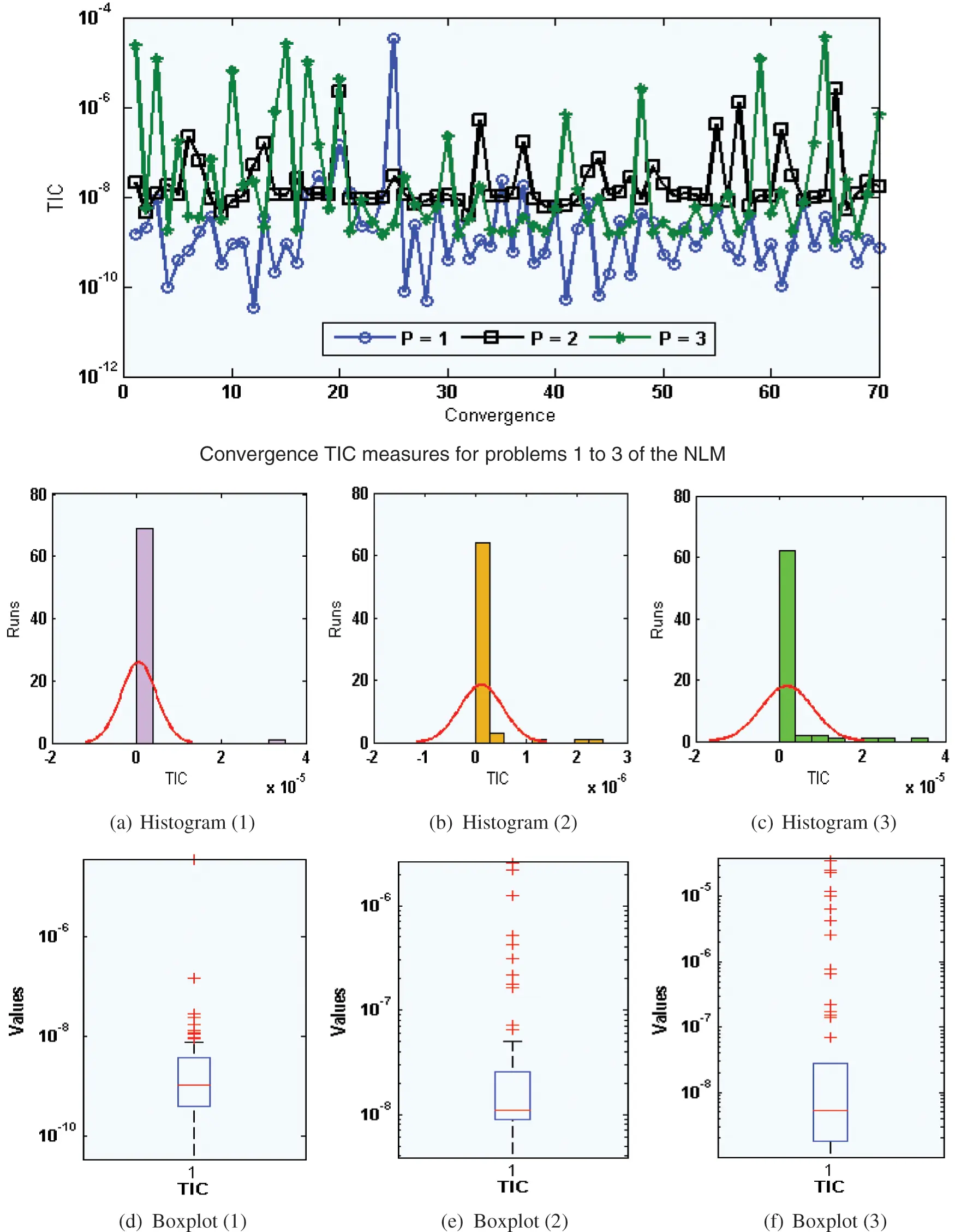Figure 4: Performances of the TIC convergence for problems 1 to 3 based NLM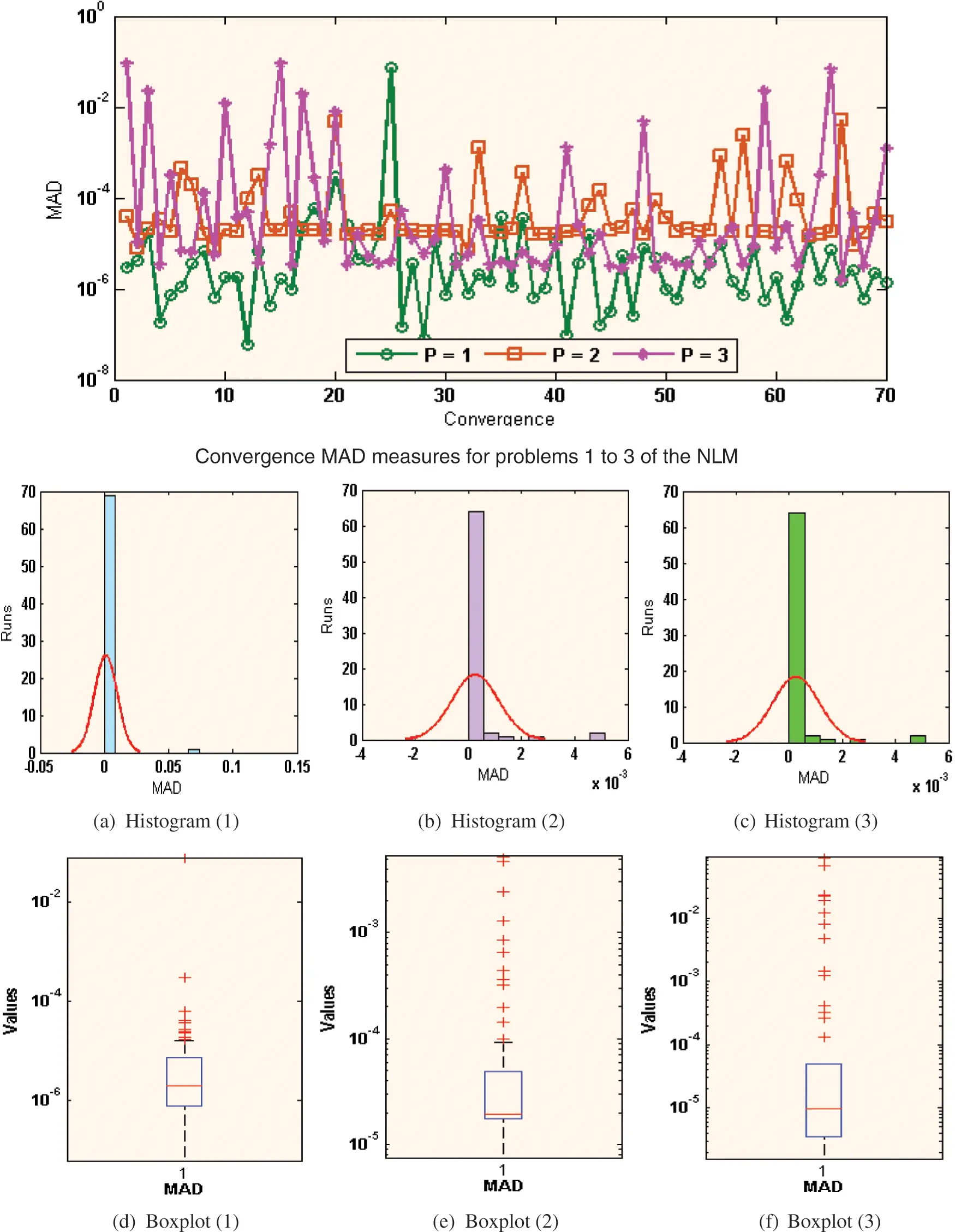Figure 5: Performances of the MAD convergence for problems 1 to 3 based NLM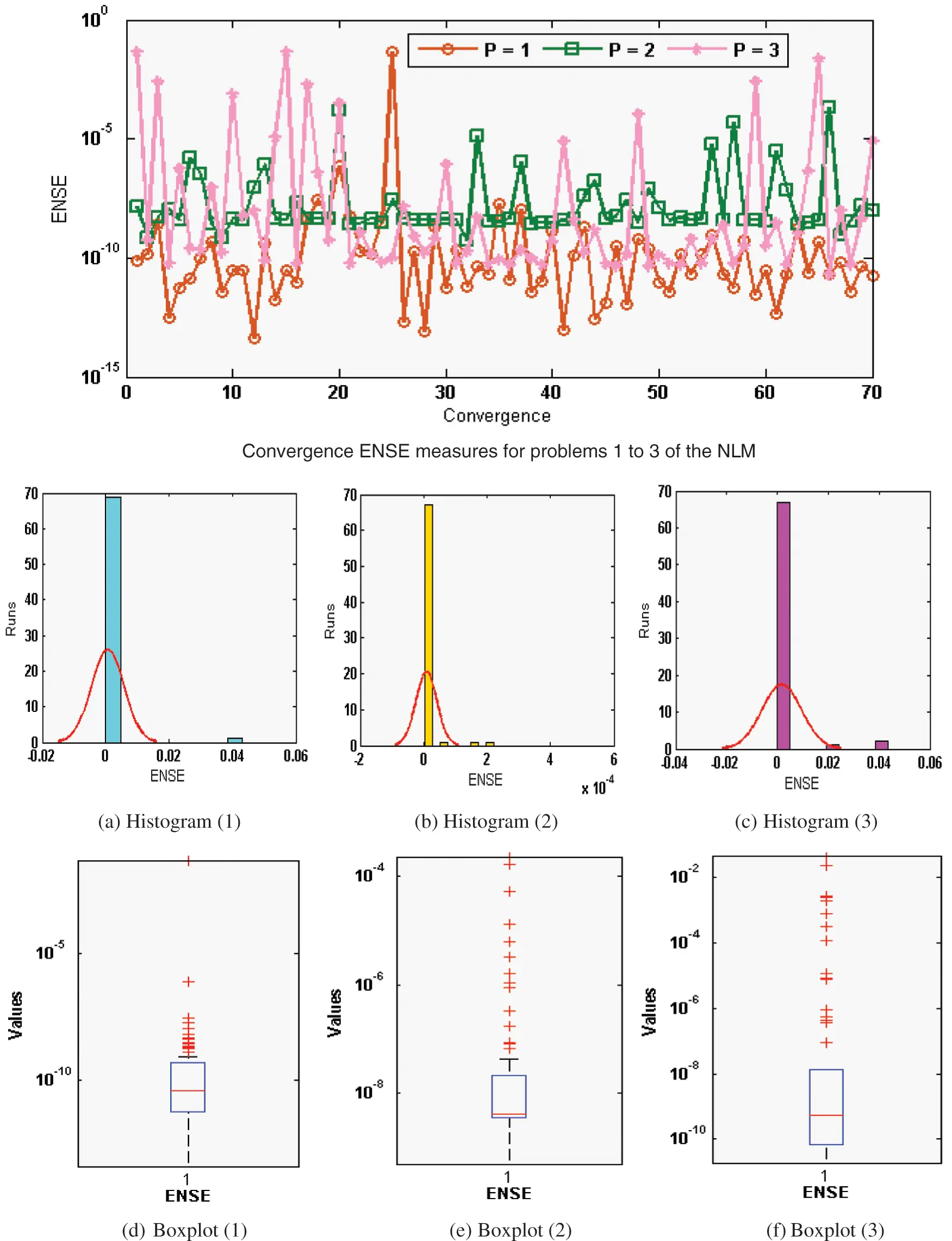Figure 6: Performances of the ENSE convergence for problems 1 to 3 based NLM

The statistical representations for Fitness (FIT), TIC, MAD and ENSE together with the histograms are provided in Figs. 36. The best FIT values are observed in Fig. 3, which are reported as 10−07–10−09, 10−08–10−11 and 10−04–10−08 for 1–3 problems. Fig. 4 shows the optimal TIC values calculated as 10−08 to 10−11 for problem 1 to 3. Fig. 5 presents the optimal MAD performances, which are observed as 10−04 to 10−08 for 1 to 3 problem. Fig. 6 illustrates the opimal ENSE performances, which are observed around 10−09–10−14 for 1st problem, 10−07–10−08 for 2nd problem and 10−04 to 10−07 for 3rd problem. In another sense, one can observe based on these performances that almost 85% of executions achieved a very reasonable and precise accuracy level of the statistical measures. The optimization tool built-in command MATLAB has been used in this study for the optimization procedure as well as other simulation studies.

In order to observe the precision as well as accuracy level of the proposed ANN along with GA-SQPS, the statistical performances based on minimum (MIN), Maximum (MAX), median (MED), Mean, S.I.R and Standard deviation (STD) are considered for 50 independent executions in Tables 24 for each problem of the NLM. The MIN operator shows the ideal results, whereas MAX values shows the bad values and the S.I.R operator. The small dependable values of the MEAN, STD, MED, MIN, S.I.R and even MAX values for the NLM show the accuracy of the stochastic performances to solve the NLM.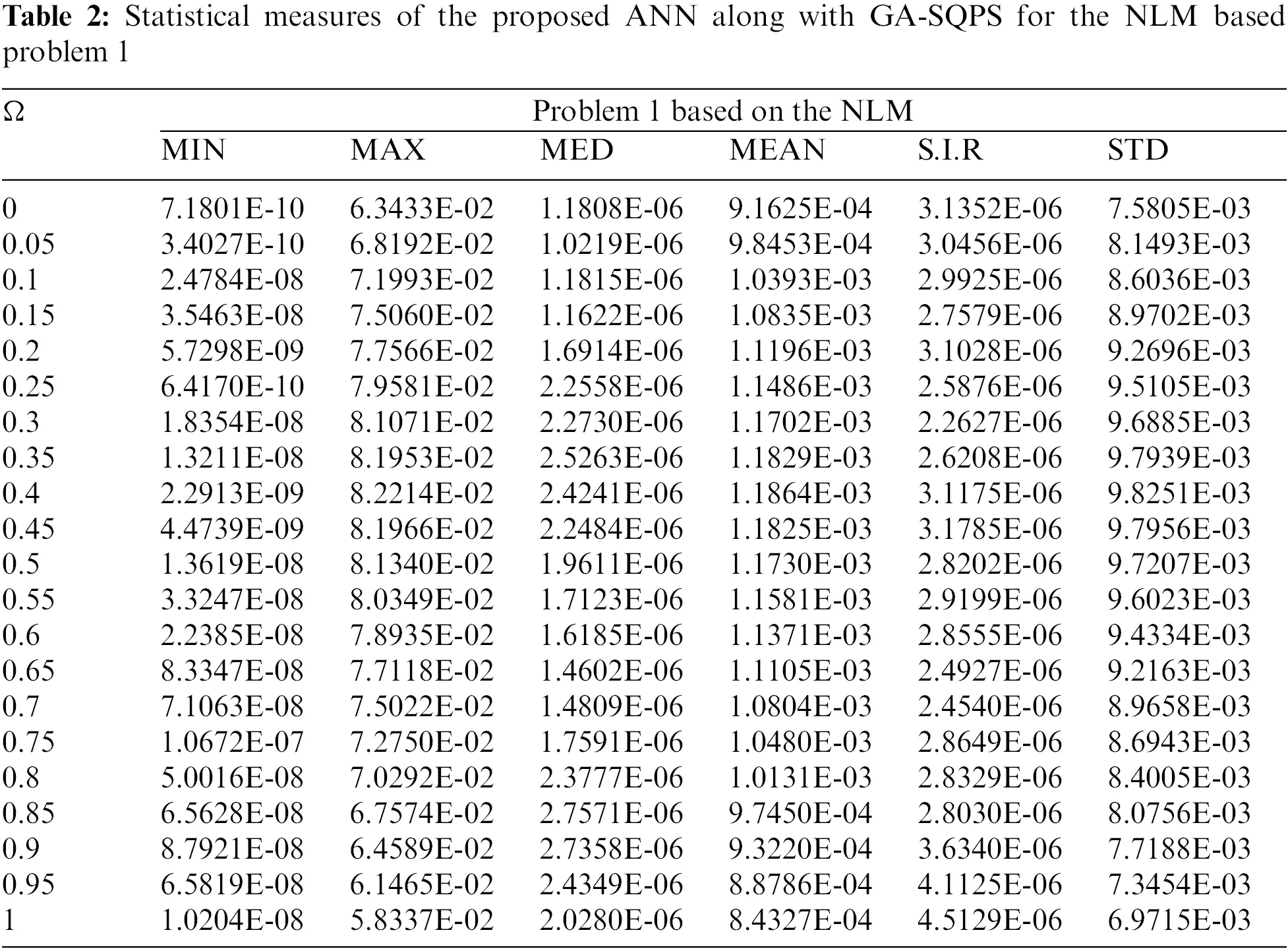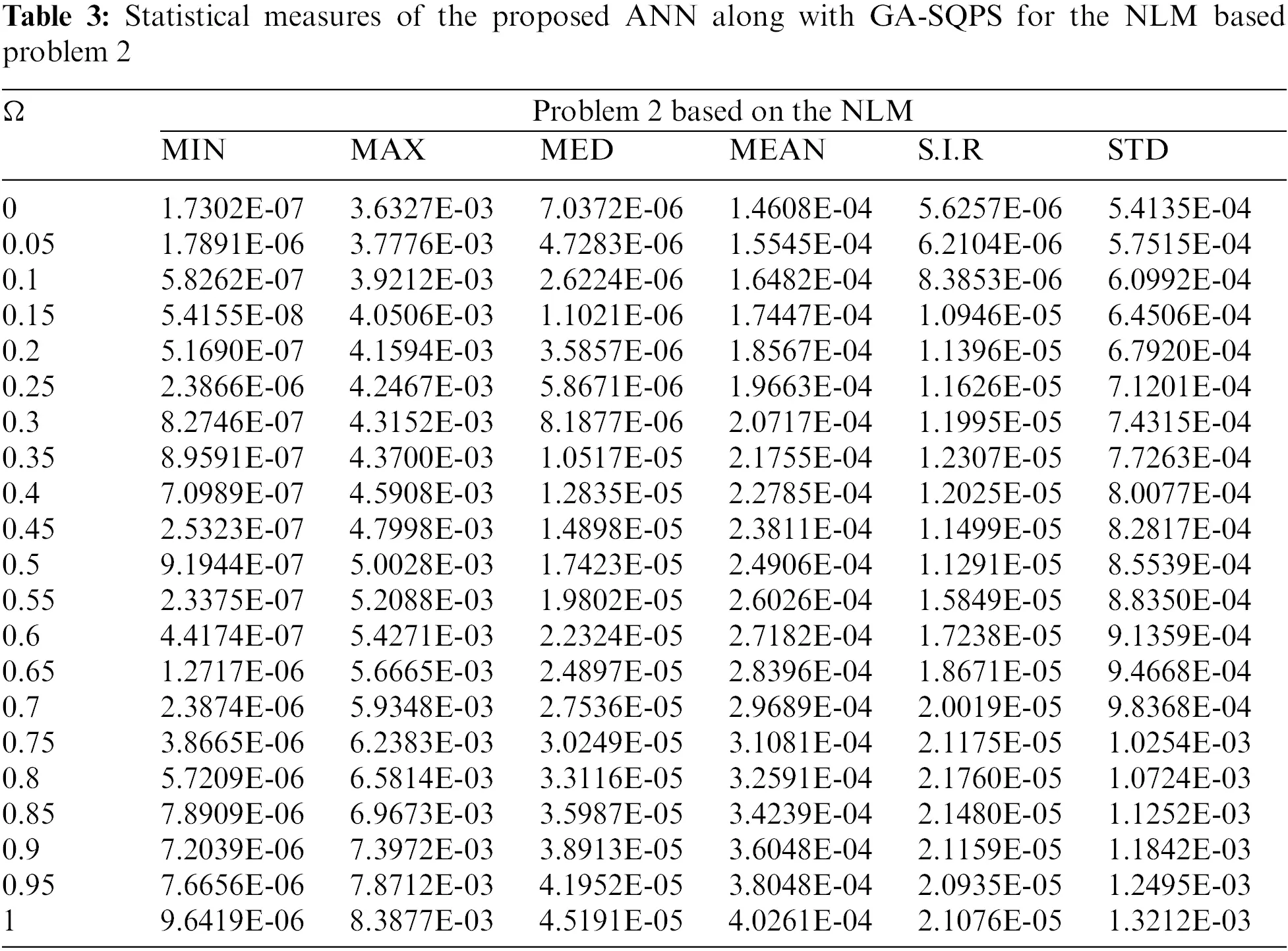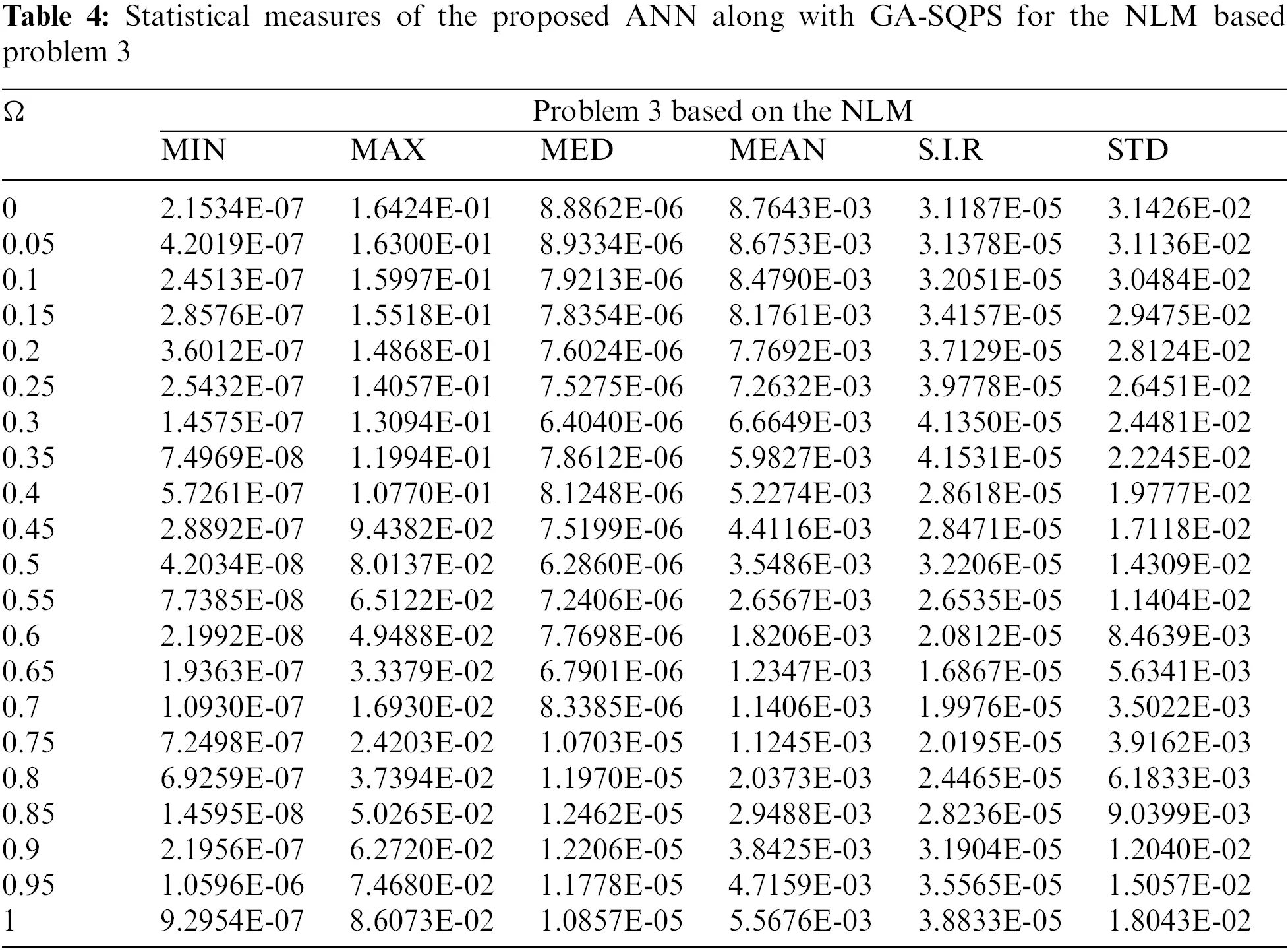The convergence measure performances are further accompanied based on the global operators of FIT, TIC, MAD and ENSE for fifty executions given in Table 5. For problems 1 to 3 based on the NLM, the MIN performances of these operators lie as 10−04–10−06, 10−03–10−04, 10−06–10−07 and 10−03–10−06. The S.I.R performances of these operators found as 10−03–10−06, 10−02–10−04, 10−06–10−07 and 10−03–10−05. These performances based global operators confirm the exactness of the designed scheme.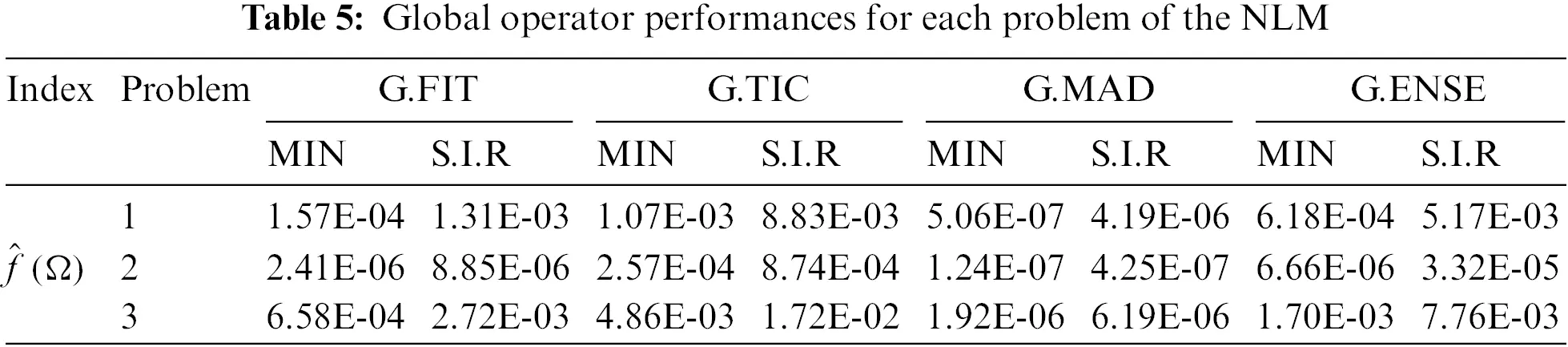The computational cost of the proposed ANN along with GA-SQPS is inspected through the parameter variation of the typical time, completed cycles/iterations and executed function count. The complexity investigations of each problem of the NLM based on the numerical measures are provided in Table 6. One can find that the average generations, executed time and function assessment lie around 113.2780, 204.7667 and 13914.3238 for each problem of the NLM. These numerical measures are provided to associate the efficiency of the ANN along with GA-SQPS.4  Conclusion

The present work is related to solving the nonlinear Liénard model numerically through the computational intelligent ANN procedures and GA-SQPS. The nonlinear Liénard differential model is observed in the generalization of a damped spring mass and the Van der Pol system. The nonlinear Liénard equations are implemented in the modeling of fluid dynamics, vacuum tube technology/radio and oscillating circuits. The optimization of the objective function has been performed through the approximation capability of ANN along with GA-SQPS. The proposed ANN along with GA-SQPS is applied for three problems based on the NLM. The correctness of the scheme is observed by comparing the proposed results with the exact solutions. The detail of the AE, performance measures through different indices, convergent plots and weight vectors have also been provided. The accurate and specific presentations of the scheme are observed as 6 to 8 decimal places of accuracy level from the exact obtainable solutions for each example of NDM. The statistical presentations of MIN, MED, MAX, S.I.R, MEAN and STD measures certify the convergence of the designed scheme for the numerical treatment of the nonlinear Liénard differential model.

In upcoming work, the NLM can be numerically treated by using the swarming optimizations scheme based on the hidden layers of the Meyer and Morlet wavelet neural networks. Moreover, these procedures can be used to solve various nonlinear and fractional order models .

Funding Statement: The authors received no specific funding for this study.

Conflicts of Interest: The authors declare that they have no conflicts of interest to report regarding the present study.

## References

1. Prajapati, R. N., Mohan, R., & Kumar, P. (2012). Numerical solution of generalized Abel’s integral equation by variational iteration method. American Journal of Computational Mathematics, 2(4), 312-315. [Google Scholar] [CrossRef]
2. Yan, L., Baskonus, H. M., Cattani, C., Gao, W. (2022). Extractions of the gravitational potential and high-frequency wave perturbation properties of nonlinear (3+1)-dimensional vakhnenko-parkes equation via novel approach. Mathematical Methods in the Applied Sciences. DOI 10.1002/mma.8726. [CrossRef]
3. Sabir, Z., Günerhan, H., & Guirao, J. L. (2020). On a new model based on third-order nonlinear multisingular functional differential equations. Mathematical Problems in Engineering, 2020, [Google Scholar] [CrossRef]
4. Abdelkawy, M. A., Sabir, Z., Guirao, J. L., & Saeed, T. (2020). Numerical investigations of a new singular second-order nonlinear coupled functional Lane–Emden model. Open Physics, 18(1), 770-778. [Google Scholar] [CrossRef]
5. Kiltu, G. G., Roba, G., & Hailu, K. (2017). Fifth order predictor-corrector method for solving quadratic riccati differential equations. International Journal of Engineering and Applied Sciences, 9(4), 51-64. [Google Scholar] [CrossRef]
6. Sabir, Z., Sakar, M. G., Yeskindirova, M., & Saldir, O. (2020). Numerical investigations to design a novel model based on the fifth order system of Emden–Fowler equations. Theoretical and Applied Mechanics Letters, 10(5), 333-342. [Google Scholar] [CrossRef]
7. Adel, W., & Sabir, Z. (2020). Solving a new design of nonlinear second-order Lane–Emden pantograph delay differential model via Bernoulli collocation method. The European Physical Journal Plus, 135(5), 427. [Google Scholar] [CrossRef]
8. Wang, B., Wang, Y., Gomez-Aguilar, J. F., Sabir, Z., & Zahoor Raja, M. A. (2022). Gudermannian neural networks to investigate the Lienard differential model. Fractals, 30(3), 2250050. [Google Scholar]
9. Zhang, Z. F., Ding, T., Huang, H. W. (1985). Qualitative theory of differential equations. Peking: Science Press.
10. Harko, T., Lobo, F. S., & Mak, M. K. (2014). A class of exact solutions of the Liénard-type ordinary nonlinear differential equation. Journal of Engineering Mathematics, 89(1), 193-205. [Google Scholar] [CrossRef]
11. Kumar, D., Agarwal, R. P., & Singh, J. (2018). A modified numerical scheme and convergence analysis for fractional model of Lienard’s equation. Journal of Computational and Applied Mathematics, 339, 405-413. [Google Scholar] [CrossRef]
12. Feng, Z. (2002). On explicit exact solutions for the Lienard equation and its applications. Physics Letters A, 293(1–2), 50-56. [Google Scholar] [CrossRef]
13. Matinfar, M., Hosseinzadeh, H., & Ghanbari, M. (2008). A numerical implementation of the variational iteration method for the Lienard equation. World Journal of Modelling and Simulation, 4(3), 205-210. [Google Scholar]
14. Matinfar, M., Mahdavi, M., & Raeisy, Z. (2011). Exact and numerical solution of Liénard’s equation by the variationalhomotopy perturbation method. Journal of Infermation and Computing Science, 6(1), 73-80. [Google Scholar]
15. Sun, J., Wang, W., & Wu, L. (2003). A note on “on explicit exact solutions for the Liénard equation and its applications”. Physics Letters A, 318(1–2), 93-101. [Google Scholar] [CrossRef]
16. Kaya, D., & El-Sayed, S. M. (2005). A numerical implementation of the decomposition method for the Lienard equation. Applied Mathematics and Computation, 171(2), 1095-1103. [Google Scholar] [CrossRef]
17. Sure, K., Aytekin, E., & Mehmet, T. A. (2016). A numerical approximation to some specific nonlinear differential equations using magnus series expansion method. New Trends in Mathematical Sciences, 4(1), 125-129. [Google Scholar] [CrossRef]
18. Matinfar, M., Bahar, S. R., & Ghasemi, M. (2012). Solving the Lienard equation by differential transform method. World Journal of Modelling and Simulation, 8(2), 142-146. [Google Scholar]
19. Kiltu, G. G., & Duressa, G. F. (2019). Accurate numerical method for Liénard nonlinear differential equations. Journal of Taibah University for Science, 13(1), 740-745. [Google Scholar] [CrossRef]
20. Sabir, Z., Raja, M. A. Z., Khalique, C. M., & Unlu, C. (2021). Neuro-evolution computing for nonlinear multi-singular system of third order Emden-Fowler equation. Mathematics and Computers in Simulation, 182, 799-812. [Google Scholar] [CrossRef]
21. Sabir, Z., Raja, M. A. Z., Guirao, J. L., & Shoaib, M. (2020). Integrated intelligent computing with neuro-swarming solver for multi-singular fourth-order nonlinear Emden–Fowler equation. Computational and Applied Mathematics, 39(4), 1-18. [Google Scholar] [CrossRef]
22. Guirao, J. L., Sabir, Z., & Saeed, T. (2020). Design and numerical solutions of a novel third-order nonlinear Emden–Fowler delay differential model. Mathematical Problems in Engineering, 2020, [Google Scholar] [CrossRef]
23. Sabir, Z., Guirao, J. L., & Saeed, T. (2021). Solving a novel designed second order nonlinear Lane–Emden delay differential model using the heuristic techniques. Applied Soft Computing, 102, 107105. [Google Scholar] [CrossRef]
24. Umar, M., Sabir, Z., Raja, M. A. Z., Amin, F., & Saeed, T. (2021). Integrated neuro-swarm heuristic with interior-point for nonlinear SITR model for dynamics of novel COVID-19. Alexandria Engineering Journal, 60(3), 2811-2824. [Google Scholar] [CrossRef]
25. Umar, M., Raja, M. A. Z., Sabir, Z., Alwabli, A. S., & Shoaib, M. (2020). A stochastic computational intelligent solver for numerical treatment of mosquito dispersal model in a heterogeneous environment. The European Physical Journal Plus, 135(7), 1-23. [Google Scholar] [CrossRef]
26. Umar, M., Sabir, Z., Amin, F., Guirao, J. L., & Raja, M. A. Z. (2020). Stochastic numerical technique for solving HIV infection model of CD4+ T cells. The European Physical Journal Plus, 135(5), 403. [Google Scholar] [CrossRef]
27. Sabir, Z., Raja, M. A. Z., Wahab, H. A., Shoaib, M., Aguilar, J. G. (2020). Integrated neuro-evolution heuristic with sequential quadratic programming for second-order prediction differential models. Numerical Methods for Partial Differential Equations. DOI 10.1002/num.22692. [CrossRef]
28. Sabir, Z., Raja, M. A. Z., Guirao, J. L., & Shoaib, M. (2021). A novel design of fractional Meyer wavelet neural networks with application to the nonlinear singular fractional Lane-Emden systems. Alexandria Engineering Journal, 60(2), 2641-2659. [Google Scholar] [CrossRef]
29. Sabir, Z., Raja, M. A. Z., Shoaib, M., & Aguilar, J. G. (2020). FMNEICS: Fractional Meyer neuro-evolution-based intelligent computing solver for doubly singular multi-fractional order Lane–Emden system. Computational and Applied Mathematics, 39(4), 1-18. [Google Scholar] [CrossRef]
30. Sabir, Z., Zahoor Raja, M. A., & Baleanu, D. (2021). Fractional mayer neuro-swarm heuristic solver for multi-fractional order doubly singular model based on Lane-Emden equation. Fractals, 29(5), [Google Scholar] [CrossRef]
31. Garai, G., & Chaudhuri, B. B. (2004). A novel genetic algorithm for automatic clustering. Pattern Recognition Letters, 25(2), 173-187. [Google Scholar] [CrossRef]
32. Zhang, C., & Wong, A. K. (1997). A genetic algorithm for multiple molecular sequence alignment. Bioinformatics, 13(6), 565-581. [Google Scholar] [CrossRef]
33. Yu, W., Li, B., Jia, H., Zhang, M., & Wang, D. (2015). Application of multi-objective genetic algorithm to optimize energy efficiency and thermal comfort in building design. Energy and Buildings, 88, 135-143. [Google Scholar] [CrossRef]
34. Adeli, H., & Cheng, N. T. (1994). Augmented lagrangian genetic algorithm for structural optimization. Journal of Aerospace Engineering, 7(1), 104-118. [Google Scholar] [CrossRef]
35. Buriol, L. S., Resende, M. G., Ribeiro, C. C., & Thorup, M. (2005). A hybrid genetic algorithm for the weight setting problem in OSPF/IS-IS routing. Networks: An International Journal, 46(1), 36-56. [Google Scholar] [CrossRef]
36. Marian, R. M., Luong, L. H., & Abhary, K. (2006). A genetic algorithm for the optimisation of assembly sequences. Computers & Industrial Engineering, 50(4), 503-527. [Google Scholar] [CrossRef]
37. Lu, J., Zhao, T., & Zhang, Y. (2008). Feature selection based-on genetic algorithm for image annotation. Knowledge-Based Systems, 21(8), 887-891. [Google Scholar] [CrossRef]
38. Kozek, T., Roska, T., & Chua, L. O. (1993). Genetic algorithm for CNN template learning. IEEE Transactions on Circuits and Systems I: Fundamental Theory and Applications, 40(6), 392-402. [Google Scholar] [CrossRef]
39. Ip, W. H., Huang, M., Yung, K. L., & Wang, D. (2003). Genetic algorithm solution for a risk-based partner selection problem in a virtual enterprise. Computers & Operations Research, 30(2), 213-231. [Google Scholar] [CrossRef]
40. Cai, J., Yan, C., Tao, Y., Lin, Y., & Wang, S. G. (2021). A novel and unified full-chip CMP model aware dummy fill insertion framework with SQP-based optimization method. IEEE Transactions on Computer-Aided Design of Integrated Circuits and Systems, 40(3), 603-607. [Google Scholar]
41. Hoppe, F., & Neitzel, I. (2021). Convergence of the SQP method for quasilinear parabolic optimal control problems. Optimization and Engineering, 22, 2039-2085. [Google Scholar]
42. Babar, M. I., Ahmad, A., & Fayyaz, S. (2020). A hybrid sine cosine algorithm with SQP for solving convex and nonconvex economic dispatch problem. Mehran University Research Journal of Engineering and Technology, 39(1), 31-46. [Google Scholar] [CrossRef]
43. Naithani, D., Chaturvedi, M., Juneja, P. K., Joshi, V. (2021). Designing of fractional order controller using SQP algorithm for industrial scale polymerization reactor. In: Advances in manufacturing and industrial engineering, pp. 445–453. Singapore: Springer.
44. Xu, B., Zhang, Y., Zhu, Z., Zhang, X., Miao, X. et al. (2020). Economic dispatch of micro-grid based on SQP—Validation and results. IOP Conference Series: Earth and Environmental Science, 446(4), 042019.
45. Wang, Y., Veeresha, P., Prakasha, D. J., Baskonus, H. M., & Gao, W. (2022). Regarding deeper properties of the fractional order Kundu-Eckhaus equation and massive thirring model. Computer Modeling in Engineering & Sciences, 133(3), 697-717. [Google Scholar] [CrossRef]
46. Veeresha, P., Ilhan, E., Prakasha, D. G., Baskonus, H. M., & Gao, W. (2021). Regarding on the fractional mathematical model of Tumour invasion and metastasis. Computer Modeling in Engineering & Sciences, 127(3), 1013-1036. [Google Scholar] [CrossRef]
47. Silambarasan, R., Baskonus, H. M., Anand, R. V., Dinakaran, M., & Balusamy, B. (2021). Longitudinal strain waves propagating in an infinitely long cylindrical rod composed of generally incompressible materials and its jacobi elliptic function solutions. Mathematics and Computers in Simulation, 182, 566-602. [Google Scholar] [CrossRef]
48. Sabir, Z. (2022). Stochastic numerical investigations for nonlinear three-species food chain system. International Journal of Biomathematics, 15(4), 2250005. [Google Scholar] [CrossRef]
49. Sabir, Z., Ali, M. R., Sadat, R. (2022). Gudermannian neural networks using the optimization procedures of genetic algorithm and active set approach for the three-species food chain nonlinear model. Journal of Ambient Intelligence and Humanized Computing, 1–10. DOI 10.1007/s12652-021-03638-3. [CrossRef]
50. Sabir, Z. (2022). Neuron analysis through the swarming procedures for the singular two-point boundary value problems arising in the theory of thermal explosion. The European Physical Journal Plus, 137(5), 638. [Google Scholar] [CrossRef]
51. Sabir, Z., Wahab, H. A., Ali, M. R., Sadat, R. (2022). Neuron analysis of the two-point singular boundary value problems arising in the thermal explosion’s theory. Neural Processing Letters, 1–28. DOI 10.1007/s11063-022-10809-6. [CrossRef]
52. Yu, Q., & Kong, S. (2021). Travelling wave solutions to the proximate equations for LWSW. Applied Mathematics and Nonlinear Sciences, 6(1), 335-346. [Google Scholar] [CrossRef]
53. Zheng, Y., Yang, L., & Sauji, F. (2021). The incomplete global GMERR algorithm for solving sylvester equation. Applied Mathematics and Nonlinear Sciences, 6(2), 1-6. [Google Scholar] [CrossRef]
54. Bulut, H., & Ismael, H. F. (2022). Exploring new features for the perturbed chen-lee-liu model via (m+1/G′)-Expansion method, proceedings of the institute of mathematics and mechanics. National Academy of Sciences of Azerbaijan, 48(1), 164-173. [Google Scholar]
55. Du, Q., Li, Y., & Pan, L. (2021). Wheelchair size and material application in human-machine system model. Applied Mathematics and Nonlinear Sciences, 6(2), 7-18. [Google Scholar] [CrossRef]
56. Akkilic, A. N., Sulaiman, T. A., & Bulut, H. (2021). Applications of the extended rational sine-cosine and sinh-cosh techniques to some nonlinear complex models arising in mathematical physics. Applied Mathematics and Nonlinear Sciences, 6(2), 19-30. [Google Scholar] [CrossRef]
57. Rasheed, S. M., Nachaoui, A., Hama, M. F., & Jabbar, A. K. (2021). Regularized and preconditioned conjugate gradient like-methods methods for polynomial approximation of an inverse Cauchy problem. Advanced Mathematical Models and Applications, 6(2), 89-105. [Google Scholar]
58. Qu, C., & Sanchez, Y. G. (2021). Nonlinear mathematical modelling of bone damage and remodelling behaviour in human femur. Applied Mathematics and Nonlinear Sciences, 6(2), 53-64. [Google Scholar] [CrossRef]
59. He, H., Song, Y., Xiao, T., Rehmab, H., & Nie, L. (2021). Design of software-defined network experimental teaching scheme based on virtualised environment. Applied Mathematics and Nonlinear Sciences, 6(2), 181-192. [Google Scholar] [CrossRef]
60. Pourghanbar, S., Manafian, J., Ranjbar, M., Aliyeva, A., & Gasimov, Y. S. (2020). An efficient alternating direction explicit method for solving a nonlinear partial differential equation. Mathematical Problems in Engineering, 2020, [Google Scholar] [CrossRef]
61. Mohit, A., & Amit, U. (2021). A modified iterative method for solving nonlinear functional equation. Applied Mathematics and Nonlinear Sciences, 6(2), 347-360. [Google Scholar] [CrossRef]
62. Bulut, H., Akkilic, A. N., & Khalid, B. J. (2021). Soliton solutions of Hirota equation and Hirota-Maccari system by the (m+1/G′)-expansion method. Advanced Mathematical Models & Applications, 6(1), 22-30. [Google Scholar]
63. Sulaiman, T. A., Bulut, H., & Baskonus, H. M. (2021). On the exact solutions to some system of complex nonlinear models. Applied Mathematics and Nonlinear Sciences, 6(1), 29-42. [Google Scholar] [CrossRef]
64. Veeresha, P. (2021). A numerical approach to the coupled atmospheric ocean model using a fractional operator. Mathematical Modelling and Numerical Simulation with Applications, 1(1), 1-10. [Google Scholar] [CrossRef]
65. Tariq, M., Ahmad, H., & Sahoo, S. K. (2021). The hermite-hadamard type inequality and its estimations via generalized convex functions of raina type. Mathematical Modelling and Numerical Simulation with Applications, 1(1), 32-43. [Google Scholar]

Yan, L., Sabir, Z., Ilhan, E., Asif, M., Gao, W. et al. (2023). Design of a Computational Heuristic to Solve the Nonlinear Liénard Differential Model. CMES-Computer Modeling in Engineering & Sciences, 136(1), 201–221.This work is licensed under a Creative Commons Attribution 4.0 International License , which permits unrestricted use, distribution, and reproduction in any medium, provided the original work is properly cited.

View

Like

## Related articles

• Candelero DJ, AJ Cristóbal, RA...
• Orona Castro F, J Medina Méndez,...
• Foroughbakhch R, RJ Ferry Sr,...
• Giselle de Moura CARPES, Viviane...
• JOÃO PAULO MARDEGAN ISSA, RODRIGO...